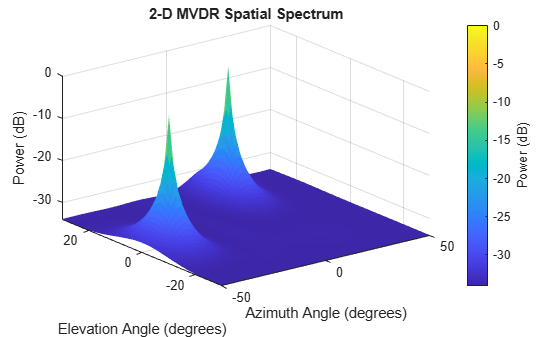Documentation

# phased.MVDREstimator2D

2-D MVDR (Capon) spatial spectrum estimator

## Description

The `MVDREstimator2D` object computes a 2-D minimum variance distortionless response (MVDR) spatial spectrum estimate. This DOA estimator is also referred to as a Capon estimator.

To estimate the spatial spectrum:

1. Define and set up your 2-D MVDR spatial spectrum estimator. See Construction.

2. Call `step` to estimate the spatial spectrum according to the properties of `phased.MVDREstimator2D`. The behavior of `step` is specific to each object in the toolbox.

### Note

Starting in R2016b, instead of using the `step` method to perform the operation defined by the System object™, you can call the object with arguments, as if it were a function. For example, ```y = step(obj,x)``` and `y = obj(x)` perform equivalent operations.

## Construction

`H = phased.MVDREstimator2D` creates a 2-D MVDR spatial spectrum estimator System object, `H`. The object estimates the signal’s spatial spectrum using a narrowband MVDR beamformer.

`H = phased.MVDREstimator2D(Name,Value)` creates object, `H`, with each specified property Name set to the specified Value. You can specify additional name-value pair arguments in any order as (`Name1`,`Value1`,...,`NameN`,`ValueN`).

## Properties

 `SensorArray` Handle to sensor array Specify the sensor array as a handle. The sensor array must be an array object in the `phased` package. The array cannot contain subarrays. Default: `phased.ULA` with default property values `PropagationSpeed` Signal propagation speed Specify the propagation speed of the signal, in meters per second, as a positive scalar. You can specify this property as single or double precision. Default: Speed of light `OperatingFrequency` System operating frequency Specify the operating frequency of the system in hertz as a positive scalar. The default value corresponds to 300 MHz. You can specify this property as single or double precision. Default: `3e8` `NumPhaseShifterBits` Number of phase shifter quantization bits The number of bits used to quantize the phase shift component of beamformer or steering vector weights. Specify the number of bits as a non-negative integer. A value of zero indicates that no quantization is performed. You can specify this property as single or double precision. Default: `0` `ForwardBackwardAveraging` Perform forward-backward averaging Set this property to `true` to use forward-backward averaging to estimate the covariance matrix for sensor arrays with conjugate symmetric array manifold. Default: `false` `AzimuthScanAngles` Azimuth scan angles (degrees) Specify the azimuth scan angles (in degrees) as a real vector. The angles must be between –180 and 180, inclusive. You must specify the angles in ascending order. You can specify this property as single or double precision. Default: `-90:90` `ElevationScanAngles` Elevation scan angles Specify the elevation scan angles (in degrees) as a real vector or scalar. The angles must be between –90 and 90, inclusive. You must specify the angles in ascending order. You can specify this property as single or double precision. Default: `0` `DOAOutputPort` Enable DOA output To obtain the signal's direction of arrival (DOA), set this property to `true` and use the corresponding output argument when invoking `step`. If you do not want to obtain the DOA, set this property to `false`. Default: `false` `NumSignals` Number of signals Specify the number of signals for DOA estimation as a positive scalar integer. This property applies when you set the `DOAOutputPort` property to `true`. You can specify this property as single or double precision. Default: `1`

## Methods

 plotSpectrum Plot spatial spectrum reset Reset states of 2-D MVDR spatial spectrum estimator object step Perform spatial spectrum estimation
Common to All System Objects
`release`

Allow System object property value changes

## Examples

expand all

Estimate the DOAs of two signals received by a 50-element URA with a rectangular lattice. The antenna operating frequency is 150 MHz. The actual direction of the first signal is −37° in azimuth and 0° in elevation. The direction of the second signal is 17° in azimuth and 20° degrees in elevation. Then, plot the spatial spectrum.

Create the arriving signals.

```fs = 8000; t = (0:1/fs:1).'; x1 = cos(2*pi*t*300); x2 = cos(2*pi*t*400); array = phased.URA('Size',[5 10],'ElementSpacing',[1 0.6]); array.Element.FrequencyRange = [100e6 300e6]; fc = 150e6; x = collectPlaneWave(array,[x1 x2],[-37 0;17 20]',fc);```

Add noise.

`noise = 0.1*(randn(size(x))+1i*randn(size(x)));`

Create the MVDR DOA estimator and estimate the DOAs.

```estimator = phased.MVDREstimator2D('SensorArray',array,... 'OperatingFrequency',fc,... 'DOAOutputPort',true,'NumSignals',2,... 'AzimuthScanAngles',-50:50,... 'ElevationScanAngles',-30:30); [~,doas] = estimator(x + noise);```

Plot the spectrum.

`plotSpectrum(estimator)`expand all

## References

 Van Trees, H. Optimum Array Processing. New York: Wiley-Interscience, 2002.

## See Also

#### Hybrid Beamforming for Massive MIMO Phased Array Systems

Download the white paper